# Making a table and plotting points given a unit rate Online Quiz

#### Excel Pivot Tables and Dashboard. Simple & Quick tutorial!

Most Popular

7 Lectures 58 mins

#### Learn HTML Tables

15 Lectures 59 mins

#### Excel Analytics - Data Analysis with Pivot-Tables and Charts

46 Lectures 2.5 hours

Following quiz provides Multiple Choice Questions (MCQs) related to Making a table and plotting points given a unit rate. You will have to read all the given answers and click over the correct answer. If you are not sure about the answer then you can check the answer using Show Answer button. You can use Next Quiz button to check new set of questions in the quiz.### Explanation

Step 1:

For the equation y = 10x, we get the following table

For the equation y = 10x, we get the following table For x = 0, y = 0, for x = 1, y = 10 and so onStep 2:

Plotting these points we get this graph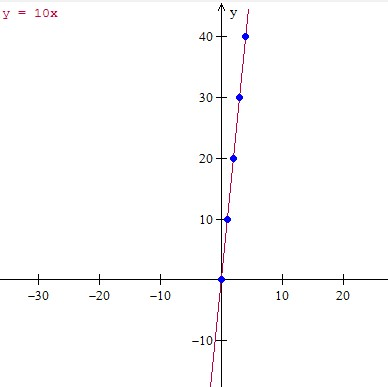### Explanation

Step 1:

For the equation y = 3x + 1, we get the following table

For x = 0, y = 1, for x = 1, y = 4 and so on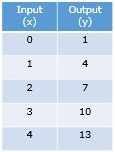Step 2:

Plotting these points we get this graph### Explanation

Step 1:

For the equation y = 8x, we get the following table

For x = 0, y = 0, for x = 1, y = 8 and so on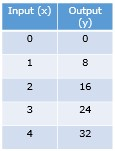Step 2:

Plotting these points we get this graph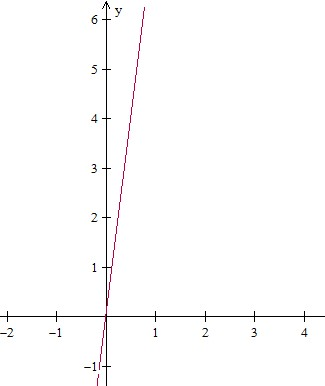### Explanation

Step 1:

For the equation y = 2x + 1, we get the following table

For x = 0, y = 1, for x = 1, y = 3 and so onStep 2:

Plotting these points we get this graph### Explanation

Step 1:

For the equation y = ($\frac{1}{2}$)x, we get the following table

For x = 0, y = 0, for x = 4, y = 2 and so onStep 2:

Plotting these points we get this graph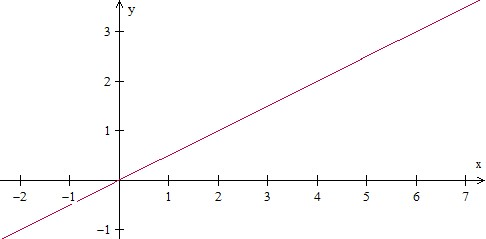### Explanation

Step 1:

For the equation y = 3x + 2, we get the following table

For x = 0, y = 2, for x = 1, y = 5 and so onStep 2:

Plotting these points we get this graph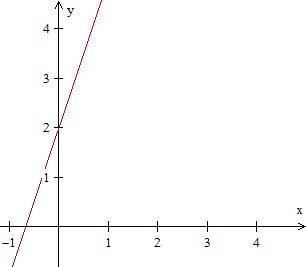### Explanation

Step 1:

For the equation y = ($\frac{1}{3}$)x, we get the following table

For x = 0, y = 0, for x = 3, y = 1 and so onStep 2:

Plotting these points we get this graph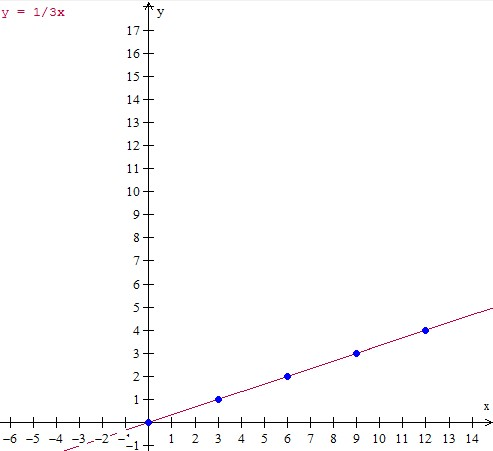### Explanation

Step 1:

For the equation y = 4x + 1, we get the following table

For x = 0, y = 1, for x = 1, y = 5 and so on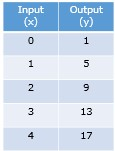Step 2:

Plotting these points we get this graph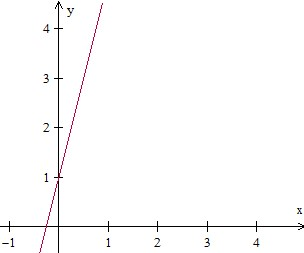### Explanation

Step 1:

For the equation y = 5x, we get the following table

For x = 0, y = 0, for x = 1, y = 5 and so on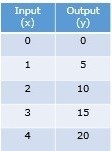Step 2:

Plotting these points we get this graph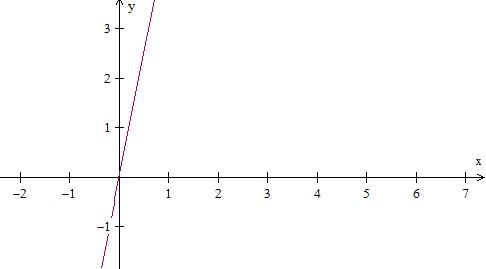### Explanation

Step 1:

For the equation y = 6x −1, we get the following table

For x = 0, y = −1, for x = 1, y = 5 and so on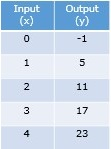Step 2:

Plotting these points we get this graphmaking_table_and_plotting_points_given_unit_rate.htm